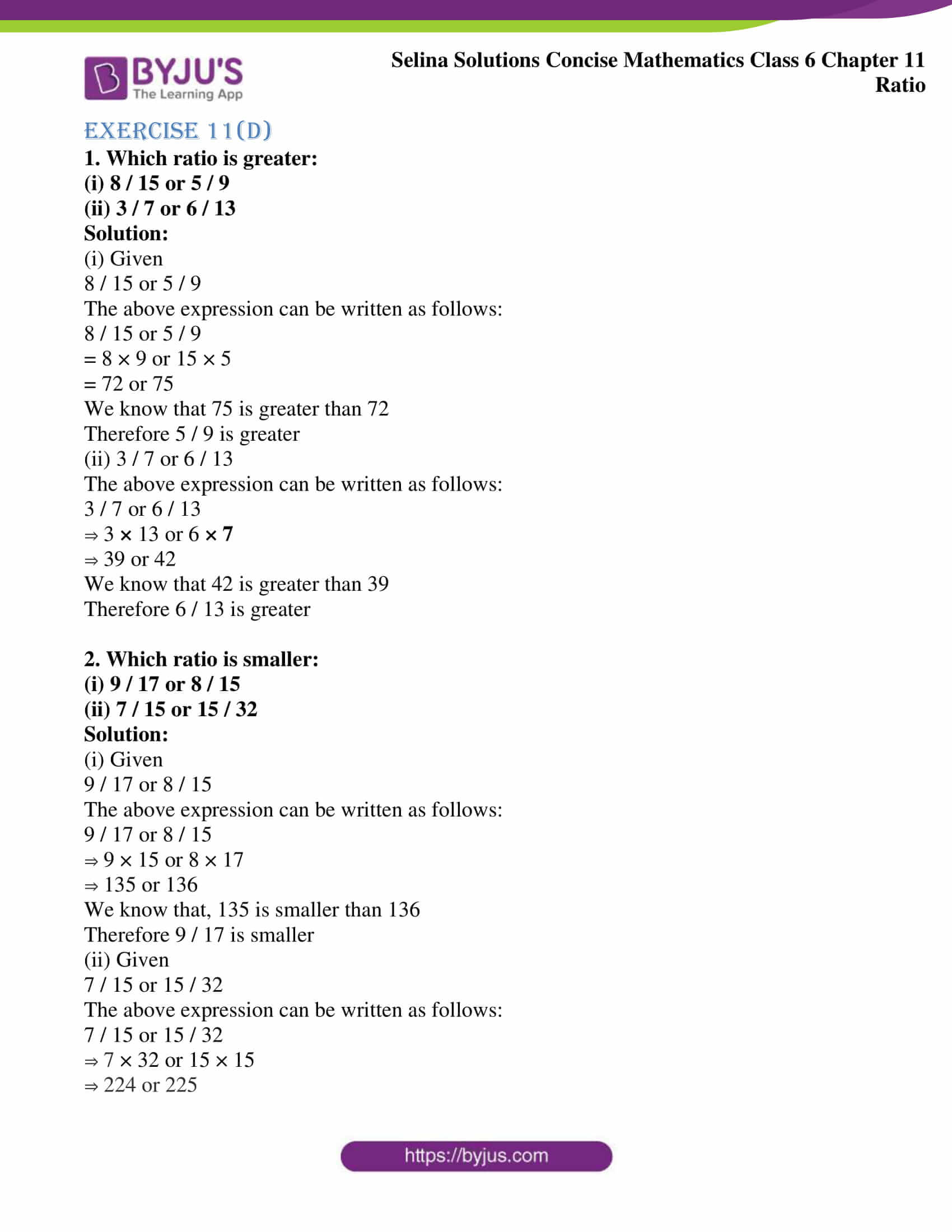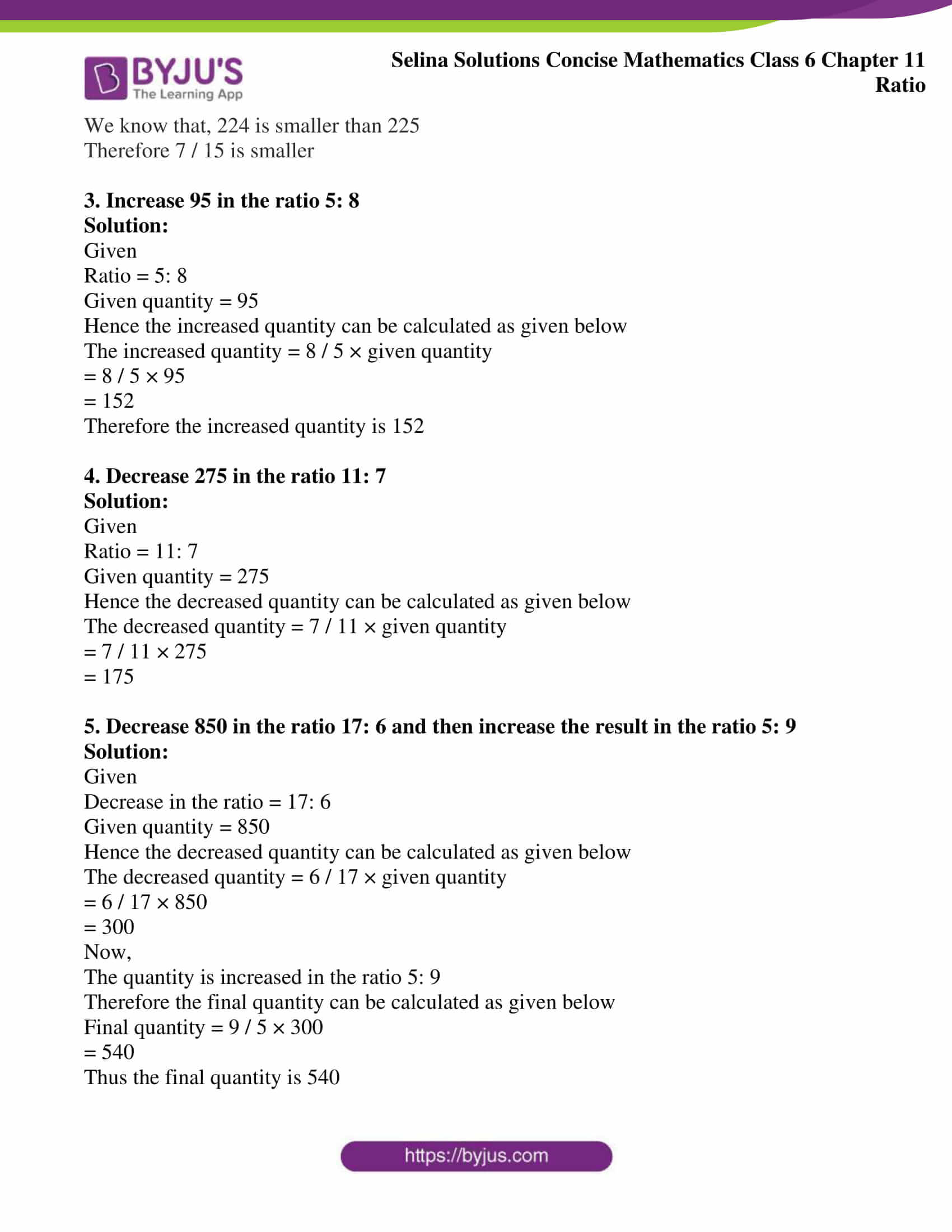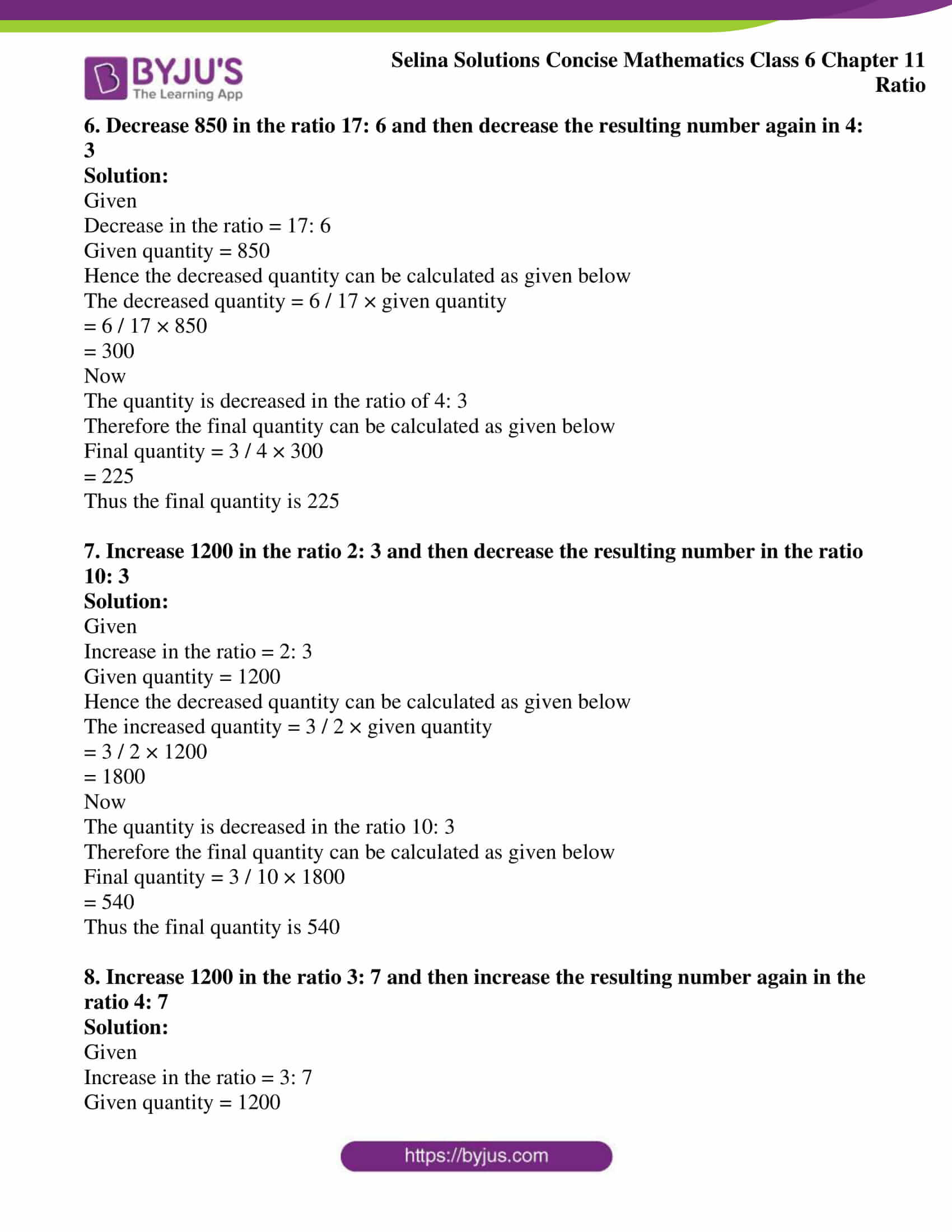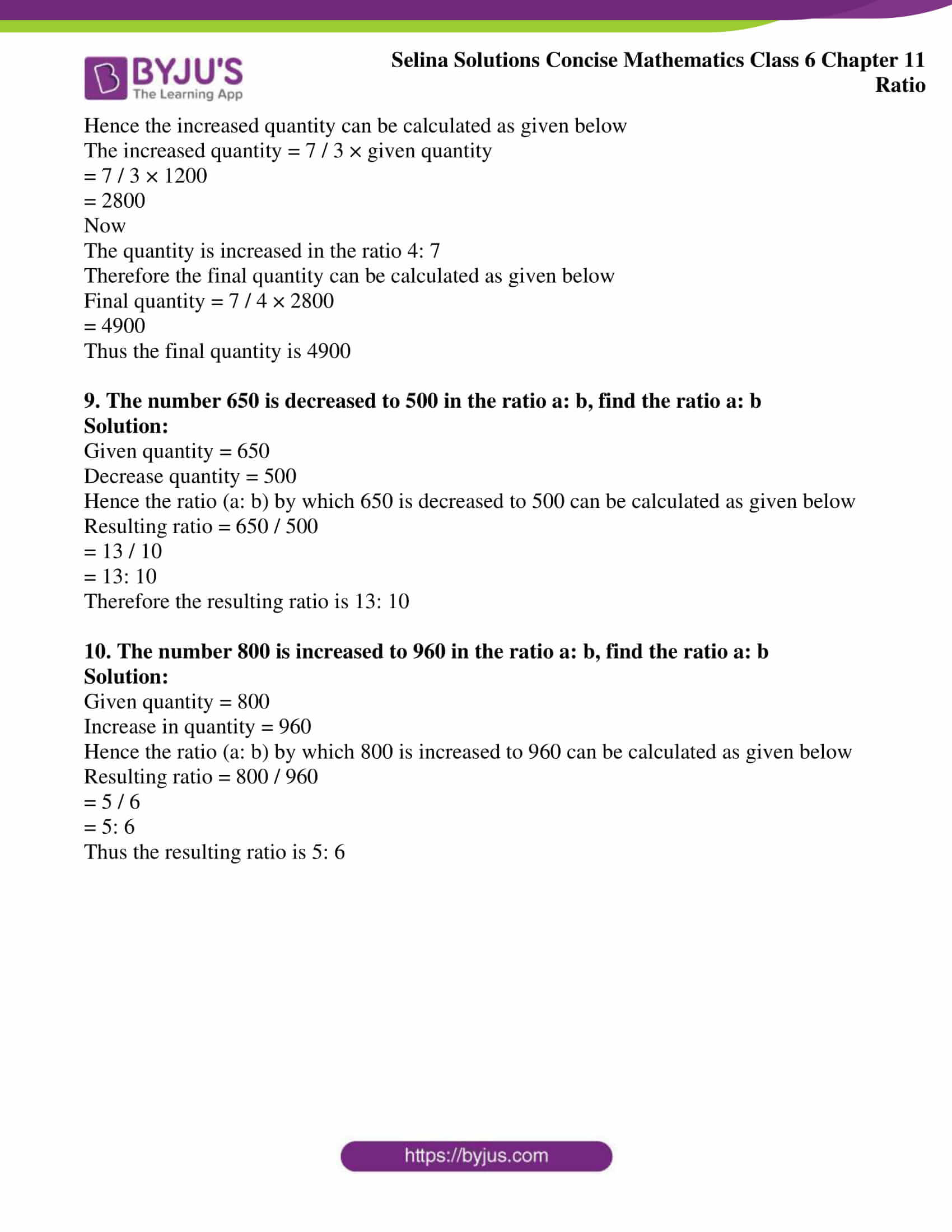# Selina Solutions Concise Mathematics Class 6 Chapter 11: Ratio Exercise 11(D)

Selina Solutions Concise Mathematics Class 6 Chapter 11 Ratio Exercise 11(D) has problems on determining the greater and smaller ratios, increase and decrease in ratios, along with illustrations. In a subject like Mathematics, students are suggested to practice the textbook questions frequently for better understanding of the topics. The solutions curated by experts, provide important formula and shortcut tricks, which help students in solving the exercise wise problems without any obstacles. Access Selina Solutions Concise Mathematics Class 6 Chapter 11 Exercise 11(D) PDF, from the available links below.

## Selina Solutions Concise Mathematics Class 6 Chapter 11 Exercise 11(D) Download PDF### Access other exercises of Selina Solutions Concise Mathematics Class 6 Chapter 11: Ratio

Exercise 11(A) Solutions

Exercise 11(B) Solutions

Exercise 11(C) Solutions

### Access Selina Solutions Concise Mathematics Class 6 Chapter 11: Ratio Exercise 11(D)

#### Exercise 11(D)

1. Which ratio is greater:

(i) 8 / 15 or 5 / 9

(ii) 3 / 7 or 6 / 13

Solution:

(i) Given

8 / 15 or 5 / 9

The above expression can be written as follows:

8 / 15 or 5 / 9

= 8 × 9 or 15 × 5

= 72 or 75

We know that 75 is greater than 72

Therefore 5 / 9 is greater

(ii) 3 / 7 or 6 / 13

The above expression can be written as follows:

3 / 7 or 6 / 13

⇒ 3 × 13 or 6 × 7

⇒ 39 or 42

We know that 42 is greater than 39

Therefore 6 / 13 is greater

2. Which ratio is smaller:

(i) 9 / 17 or 8 / 15

(ii) 7 / 15 or 15 / 32

Solution:

(i) Given

9 / 17 or 8 / 15

The above expression can be written as follows:

9 / 17 or 8 / 15

⇒ 9 × 15 or 8 × 17

⇒ 135 or 136

We know that, 135 is smaller than 136

Therefore 9 / 17 is smaller

(ii) Given

7 / 15 or 15 / 32

The above expression can be written as follows:

7 / 15 or 15 / 32

⇒ 7 × 32 or 15 × 15

⇒ 224 or 225

We know that, 224 is smaller than 225

Therefore 7 / 15 is smaller

3. Increase 95 in the ratio 5: 8

Solution:

Given

Ratio = 5: 8

Given quantity = 95

Hence the increased quantity can be calculated as given below

The increased quantity = 8 / 5 × given quantity

= 8 / 5 × 95

= 152

Therefore the increased quantity is 152

4. Decrease 275 in the ratio 11: 7

Solution:

Given

Ratio = 11: 7

Given quantity = 275

Hence the decreased quantity can be calculated as given below

The decreased quantity = 7 / 11 × given quantity

= 7 / 11 × 275

= 175

5. Decrease 850 in the ratio 17: 6 and then increase the result in the ratio 5: 9

Solution:

Given

Decrease in the ratio = 17: 6

Given quantity = 850

Hence the decreased quantity can be calculated as given below

The decreased quantity = 6 / 17 × given quantity

= 6 / 17 × 850

= 300

Now,

The quantity is increased in the ratio 5: 9

Therefore the final quantity can be calculated as given below

Final quantity = 9 / 5 × 300

= 540

Thus the final quantity is 540

6. Decrease 850 in the ratio 17: 6 and then decrease the resulting number again in 4: 3

Solution:

Given

Decrease in the ratio = 17: 6

Given quantity = 850

Hence the decreased quantity can be calculated as given below

The decreased quantity = 6 / 17 × given quantity

= 6 / 17 × 850

= 300

Now

The quantity is decreased in the ratio of 4: 3

Therefore the final quantity can be calculated as given below

Final quantity = 3 / 4 × 300

= 225

Thus the final quantity is 225

7. Increase 1200 in the ratio 2: 3 and then decrease the resulting number in the ratio 10: 3

Solution:

Given

Increase in the ratio = 2: 3

Given quantity = 1200

Hence the decreased quantity can be calculated as given below

The increased quantity = 3 / 2 × given quantity

= 3 / 2 × 1200

= 1800

Now

The quantity is decreased in the ratio 10: 3

Therefore the final quantity can be calculated as given below

Final quantity = 3 / 10 × 1800

= 540

Thus the final quantity is 540

8. Increase 1200 in the ratio 3: 7 and then increase the resulting number again in the ratio 4: 7

Solution:

Given

Increase in the ratio = 3: 7

Given quantity = 1200

Hence the increased quantity can be calculated as given below

The increased quantity = 7 / 3 × given quantity

= 7 / 3 × 1200

= 2800

Now

The quantity is increased in the ratio 4: 7

Therefore the final quantity can be calculated as given below

Final quantity = 7 / 4 × 2800

= 4900

Thus the final quantity is 4900

9. The number 650 is decreased to 500 in the ratio a: b, find the ratio a: b

Solution:

Given quantity = 650

Decrease quantity = 500

Hence the ratio (a: b) by which 650 is decreased to 500 can be calculated as given below

Resulting ratio = 650 / 500

= 13 / 10

= 13: 10

Therefore the resulting ratio is 13: 10

10. The number 800 is increased to 960 in the ratio a: b, find the ratio a: b

Solution:

Given quantity = 800

Increase in quantity = 960

Hence the ratio (a: b) by which 800 is increased to 960 can be calculated as given below

Resulting ratio = 800 / 960

= 5 / 6

= 5: 6

Thus the resulting ratio is 5: 6